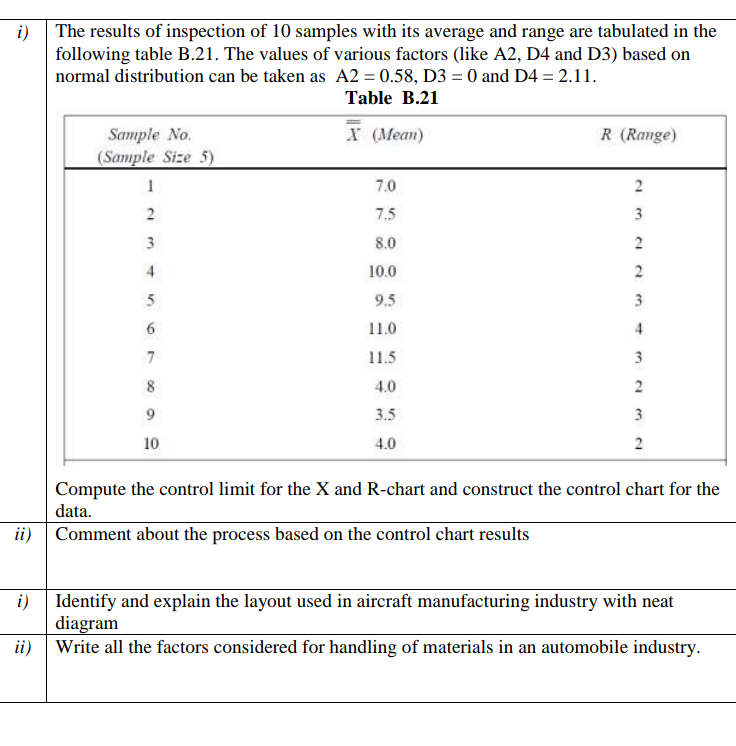# The results of inspection of 10 samples with its average and range are tabulated in the following table B.21. The values of various factors (like A2, D4 and D3) based on normal distribution can be taken as A2 = 0.58, D3 = 0 and D4 = 2.11.

Question-AnswerCategory: Manufacturing/ProductionThe results of inspection of 10 samples with its average and range are tabulated in the following table B.21. The values of various factors (like A2, D4 and D3) based on normal distribution can be taken as A2 = 0.58, D3 = 0 and D4 = 2.11.

The results of inspection of 10 samples with its average and range are tabulated in the following table B.21. The values of various factors (like A2, D4 and D3) based on normal distribution can be taken as A2 = 0.58, D3 = 0 and D4 = 2.11.manufacturing operation management